# class TGeoManager: public TNamed

``` General architecture

The new ROOT geometry package is a tool designed for building, browsing,
tracking and visualizing a detector geometry. The code is independent from
other external MC for simulation, therefore it does not contain any
constraints related to physics. However, the package defines a number of
hooks for tracking, such as media, materials, magnetic field or track state flags,
in order to allow interfacing to tracking MC's. The final goal is to be
able to use the same geometry for several purposes, such as tracking,
reconstruction or visualization, taking advantage of the ROOT features
related to bookkeeping, I/O, histograming, browsing and GUI's.

The geometrical modeler is the most important component of the package and
it provides answers to the basic questions like "Where am I ?" or "How far
from the next boundary ?", but also to more complex ones like "How far from
the closest surface ?" or "Which is the next crossing along a helix ?".

The architecture of the modeler is a combination between a GEANT-like
containment scheme and a normal CSG binary tree at the level of shapes. An
important common feature of all detector geometry descriptions is the
mother-daughter concept. This is the most natural approach when tracking
is concerned and imposes a set of constraints to the way geometry is defined.
Constructive solid geometry composition is used only in order to create more
complex shapes from an existing set of primitives through boolean operations.
This feature is not implemented yet but in future full definition of boolean
expressions will be supported.

Practically every geometry defined in GEANT style can be mapped by the modeler.
The basic components used for building the logical hierarchy of the geometry
are called "volumes" and "nodes". Volumes (sometimes called "solids") are fully
defined geometrical objects having a given shape and medium and possibly
containing a list of nodes. Nodes represent just positioned instances of volumes
inside a container volume and they are not directly defined by user. They are
automatically created as a result of adding one volume inside other or dividing
a volume. The geometrical transformation hold by nodes is always defined with
respect to their mother (relative positioning). Reflection matrices are allowed.
All volumes have to be fully aware of their containees when the geometry is
closed. They will build aditional structures (voxels) in order to fasten-up
the search algorithms. Finally, nodes can be regarded as bidirectional links
between containers and containees objects.

The structure defined in this way is a graph structure since volumes are
replicable (same volume can become daughter node of several other volumes),
every volume becoming a branch in this graph. Any volume in the logical graph
can become the actual top volume at run time (see TGeoManager::SetTopVolume()).
All functionalities of the modeler will behave in this case as if only the
corresponding branch starting from this volume is the registered geometry.

```
/*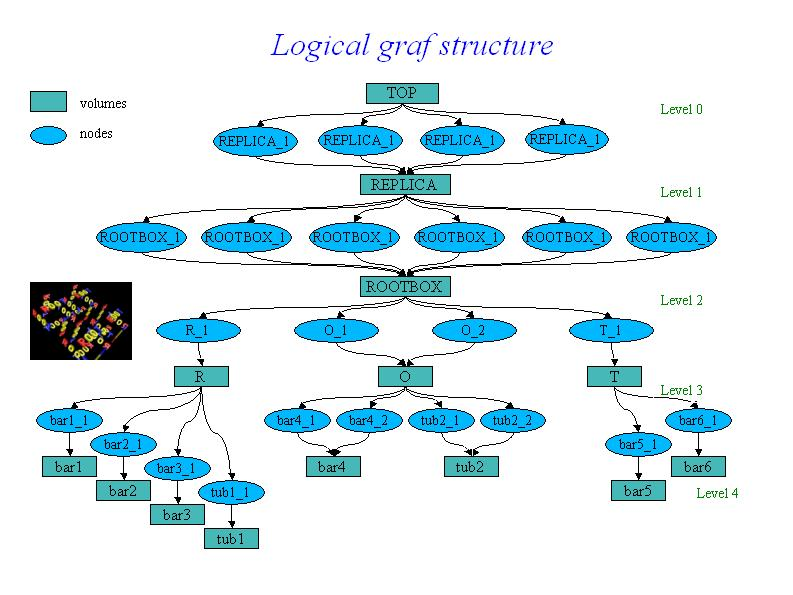*/
```
A given volume can be positioned several times in the geometry. A volume
can be divided according default or user-defined patterns, creating automatically
the list of division nodes inside. The elementary volumes created during the
dividing process follow the same scheme as usual volumes, therefore it is possible
to position further geometrical structures inside or to divide them further more
(see TGeoVolume::Divide()).

The primitive shapes supported by the package are basically the GEANT3
shapes (see class TGeoShape), arbitrary wedges with eight vertices on two parallel
planes. All basic primitives inherits from class TGeoBBox since the bounding box
of a solid is essential for the tracking algorithms. They also implement the
virtual methods defined in the virtual class TGeoShape (point and segment
classification). User-defined primitives can be direcly plugged into the modeler
provided that they override these methods. Composite shapes will be soon supported
by the modeler. In order to build a TGeoCompositeShape, one will have to define
first the primitive components. The object that handle boolean
operations among components is called TGeoBoolCombinator and it has to be
constructed providing a string boolean expression between the components names.

Example for building a simple geometry :

```
rootgeom.C //
```                                                             //

```
/*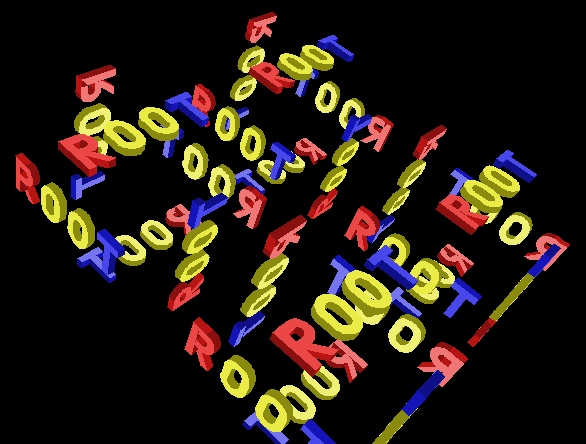*/
```

TGeoManager - the manager class for the geometry package.

TGeoManager class is embedding all the API needed for building and tracking
a geometry. It defines a global pointer (gGeoManager) in order to be fully
accessible from external code. The mechanism of handling multiple geometries
at the same time will be soon implemented.

TGeoManager is the owner of all geometry objects defined in a session,
therefore users must not try to control their deletion. It contains lists of
media, materials, transformations, shapes and volumes. Logical nodes (positioned
volumes) are created and destroyed by the TGeoVolume class. Physical
nodes and their global transformations are subjected to a caching mechanism
due to the sometimes very large memory requirements of logical graph expansion.
The caching mechanism is triggered by the total number of physical instances
of volumes and the cache manager is a client of TGeoManager. The manager class
also controls the painter client. This is linked with ROOT graphical libraries
loaded on demand in order to control visualization actions.

Rules for building a valid geometry

A given geometry can be built in various ways, but there are mandatory steps
that have to be followed in order to be validated by the modeler. There are
general rules : volumes needs media and shapes in order to be created,
both container an containee volumes must be created before linking them together,
and the relative transformation matrix must be provided. All branches must
have an upper link point otherwise they will not be considered as part of the
geometry. Visibility or tracking properties of volumes can be provided both
at build time or after geometry is closed, but global visualization settings
(see TGeoPainter class) should not be provided at build time, otherwise the
drawing package will be loaded. There is also a list of specific rules :
positioned daughters should not extrude their mother or intersect with sisters
unless this is specified (see TGeoVolume::AddNodeOverlap()), the top volume
(containing all geometry tree) must be specified before closing the geometry
and must not be positioned - it represents the global reference frame. After
building the full geometry tree, the geometry must be closed
(see TGeoManager::CloseGeometry()). Voxelization can be redone per volume after
this process.

Below is the general scheme of the manager class.

```
/*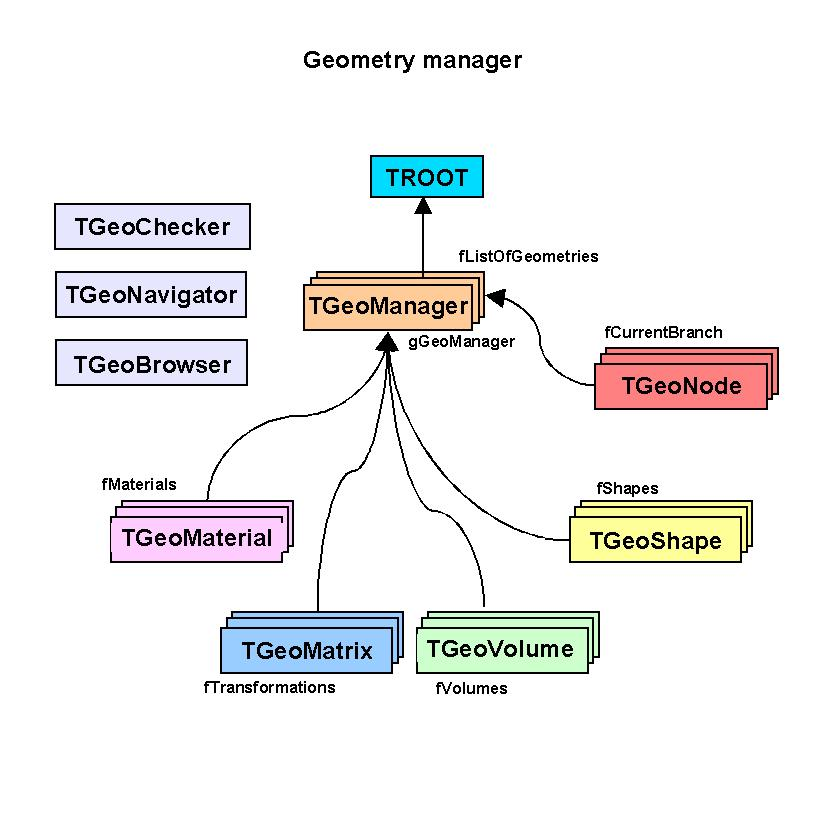*/
```
An interactive session

Provided that a geometry was successfully built and closed (for instance the
previous example \$ROOTSYS/tutorials/geom/rootgeom.C ), the manager class will register
itself to ROOT and the logical/physical structures will become immediately browsable.
The ROOT browser will display starting from the geometry folder : the list of
transformations and media, the top volume and the top logical node. These last
two can be fully expanded, any intermediate volume/node in the browser being subject
of direct access context menu operations (right mouse button click). All user
utilities of classes TGeoManager, TGeoVolume and TGeoNode can be called via the

```
/*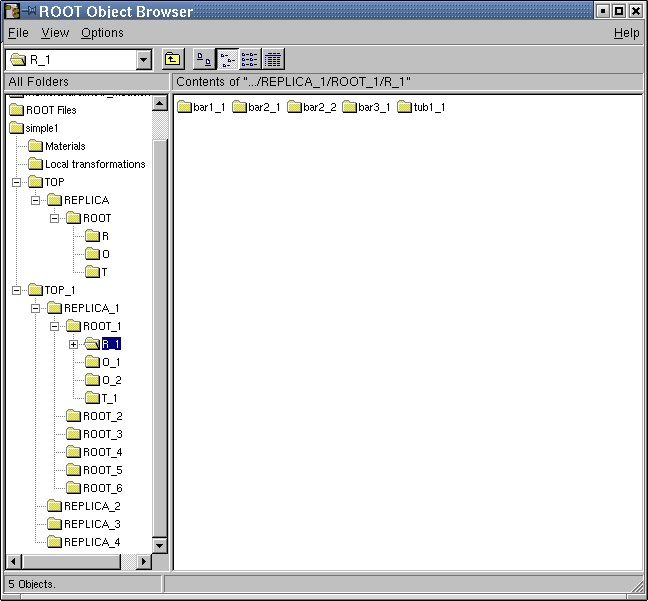*/
```
--- Drawing the geometry

Any logical volume can be drawn via TGeoVolume::Draw() member function.
This can be direcly accessed from the context menu of the volume object
directly from the browser.
There are several drawing options that can be set with
TGeoManager::SetVisOption(Int_t opt) method :
opt=0 - only the content of the volume is drawn, N levels down (default N=3).
This is the default behavior. The number of levels to be drawn can be changed
via TGeoManager::SetVisLevel(Int_t level) method.

```
/*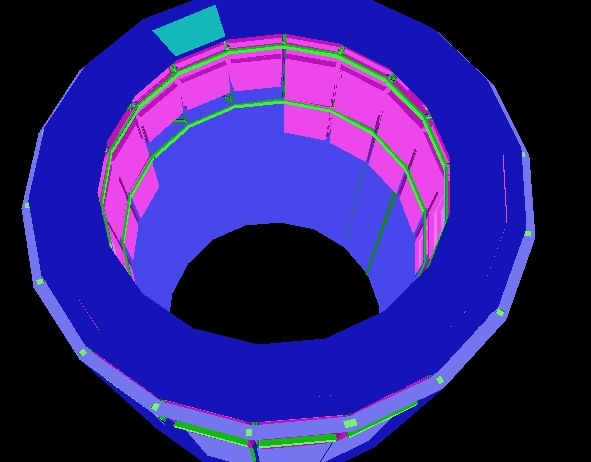*/
```
opt=1 - the final leaves (e.g. daughters with no containment) of the branch
starting from volume are drawn down to the current number of levels.
WARNING : This mode is memory consuming
depending of the size of geometry, so drawing from top level within this mode
should be handled with care for expensive geometries. In future there will be
a limitation on the maximum number of nodes to be visualized.

```
/*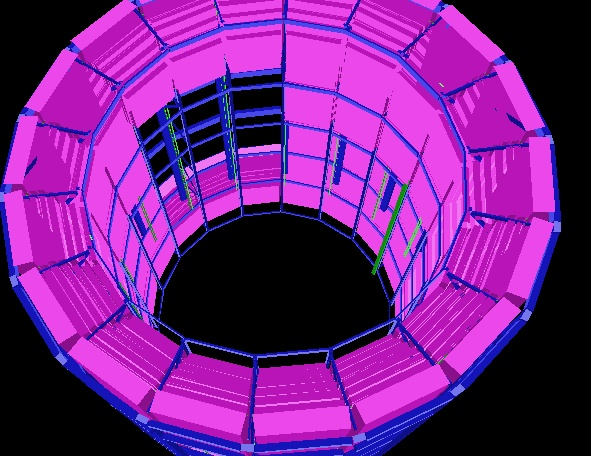*/
```
opt=2 - only the clicked volume is visualized. This is automatically set by
TGeoVolume::DrawOnly() method
opt=3 - only a given path is visualized. This is automatically set by
TGeoVolume::DrawPath(const char *path) method

The current view can be exploded in cartesian, cylindrical or spherical
coordinates :
TGeoManager::SetExplodedView(Int_t opt). Options may be :
- 0  - default (no bombing)
- 1  - cartesian coordinates. The bomb factor on each axis can be set with
TGeoManager::SetBombX(Double_t bomb) and corresponding Y and Z.
- 2  - bomb in cylindrical coordinates. Only the bomb factors on Z and R
are considered

```
/*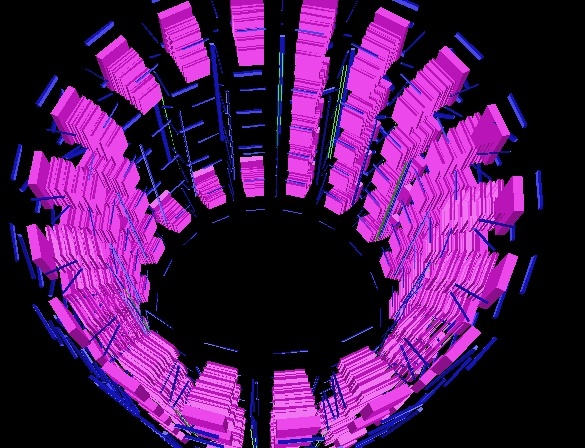*/
```
- 3  - bomb in radial spherical coordinate : TGeoManager::SetBombR()

Volumes themselves support different visualization settings :
- TGeoVolume::SetVisibility() : set volume visibility.
- TGeoVolume::VisibleDaughters() : set daughters visibility.
All these actions automatically updates the current view if any.

--- Checking the geometry

Several checking methods are accessible from the volume context menu. They
generally apply only to the visible parts of the drawn geometry in order to
ease geometry checking, and their implementation is in the TGeoChecker class
from the painting package.

1. Checking a given point.
Can be called from TGeoManager::CheckPoint(Double_t x, Double_t y, Double_t z).
This method is drawing the daughters of the volume containing the point one
level down, printing the path to the deepest physical node holding this point.
It also computes the closest distance to any boundary. The point will be drawn
in red.

```
/*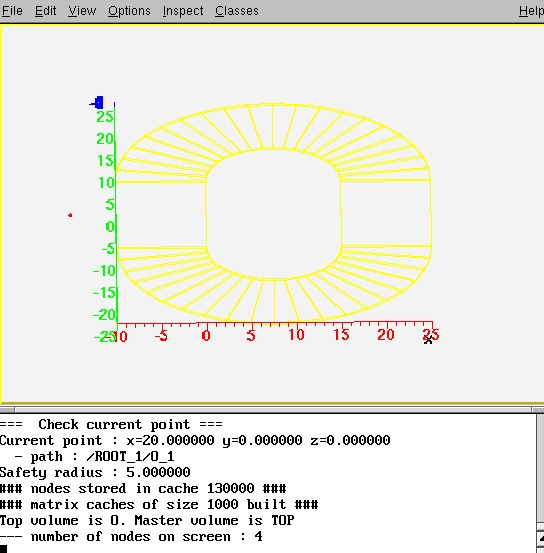*/
```
2. Shooting random points.
Can be called from TGeoVolume::RandomPoints() (context menu function) and
it will draw this volume with current visualization settings. Random points
are generated in the bounding box of the top drawn volume. The points are
classified and drawn with the color of their deepest container. Only points
in visible nodes will be drawn.

```
/**/
```

3. Raytracing.
Can be called from TGeoVolume::RandomRays() (context menu of volumes) and
will shoot rays from a given point in the local reference frame with random
directions. The intersections with displayed nodes will appear as segments
having the color of the touched node. Drawn geometry will be then made invisible
in order to enhance rays.

```
/*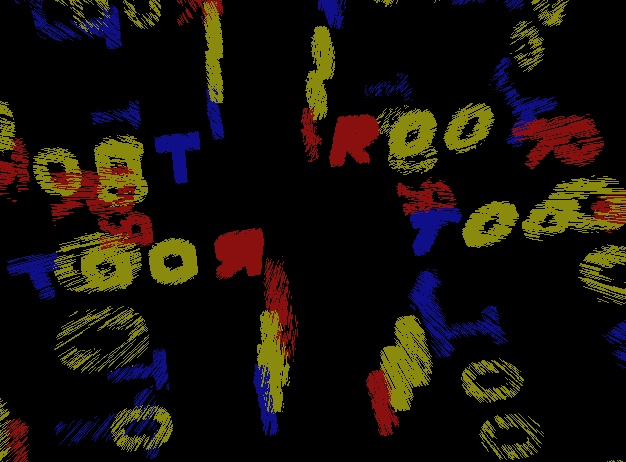*/

## Function Members (Methods)

public:
protected:
 TGeoManager(const TGeoManager&) virtual void TObject::DoError(int level, const char* location, const char* fmt, va_list va) const void TObject::MakeZombie() TGeoManager& operator=(const TGeoManager&)
private:
 void Init() Bool_t InitArrayPNE() const Bool_t InsertPNEId(Int_t uid, Int_t ientry) Bool_t IsLoopingVolumes() const void SetLoopVolumes(Bool_t flag = kTRUE) void UpdateElements() void Voxelize(Option_t* option = 0)

## Data Members

public:
 enum TObject::EStatusBits { kCanDelete kMustCleanup kObjInCanvas kIsReferenced kHasUUID kCannotPick kNoContextMenu kInvalidObject }; enum TObject::[unnamed] { kIsOnHeap kNotDeleted kZombie kBitMask kSingleKey kOverwrite kWriteDelete };
protected:
 TString TNamed::fName object identifier TString TNamed::fTitle object title static Bool_t fgLock ! Lock preventing a second geometry to be loaded static Int_t fgMaxDaughters ! Maximum number of daughters static Int_t fgMaxLevel ! Maximum level in geometry static Int_t fgMaxXtruVert ! Maximum number of Xtru vertices static Int_t fgVerboseLevel ! Verbosity level for Info messages (no IO).
private:

## Class Charts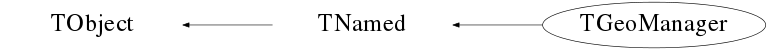## Function documentation

``` Default constructor.
```
TGeoManager(const char* name, const char* title)
``` Constructor.
```
void Init()
``` Initialize manager class.
```
TGeoManager(const TGeoManager& )
```copy constructor
```
TGeoManager& operator=(const TGeoManager& )
```assignment operator
```

```   Destructor
```
``` Add a material to the list. Returns index of the material in list.
```
``` Add an illegal overlap/extrusion to the list.
```
``` Add a matrix to the list. Returns index of the matrix in list.
```
``` Add a shape to the list. Returns index of the shape in list.
```
Int_t AddTrack(Int_t id, Int_t pdgcode, TObject* particle = 0)
``` Add a track to the list of tracks. Use this for primaries only. For secondaries,
add them to the parent track. The method create objects that are registered
to the analysis manager but have to be cleaned-up by the user via ClearTracks().
```
``` Add a track to the list of tracks
```
TVirtualGeoTrack * MakeTrack(Int_t id, Int_t pdgcode, TObject* particle)
``` Makes a primary track but do not attach it to the list of tracks. The track
can be attached as daughter to another one with TVirtualGeoTrack::AddTrack
```
``` Add a volume to the list. Returns index of the volume in list.
```

``` Add a navigator in the list of navigators. If it is the first one make it
current navigator.
```
TGeoNavigator * GetCurrentNavigator() const
``` Returns current navigator for the calling thread.
```

``` Get list of navigators for the calling thread.
```

``` Switch to another existing navigator for the calling thread.
```
void SetNavigatorsLock(Bool_t flag)
``` Set the lock for navigators.
```
void ClearNavigators()
``` Clear all navigators.
```
void RemoveNavigator(const TGeoNavigator* nav)
``` Clear a single navigator.
```
``` Set maximum number of threads for navigation.
```
``` Create thread private data for all geometry objects.
```
``` Clear the current map of threads. This will be filled again by the calling
```

``` Translates the current thread id to an ordinal number. This can be used to
manage data which is pspecific for a given thread.
static __thread Int_t tid = -1;
if (tid > -1) return tid;
```
void Browse(TBrowser* b)
``` Describe how to browse this object.
```
void Edit(Option_t* option = "")
``` Append a pad for this geometry.
```
void SetVisibility(TObject* obj, Bool_t vis)
``` Set visibility for a volume.
```
void BombTranslation(const Double_t* tr, Double_t* bombtr)
``` Get the new 'bombed' translation vector according current exploded view mode.
```
void UnbombTranslation(const Double_t* tr, Double_t* bombtr)
``` Get the new 'unbombed' translation vector according current exploded view mode.
```
void DoBackupState()
``` Backup the current state without affecting the cache stack.
```
void DoRestoreState()
``` Restore a backed-up state without affecting the cache stack.
```
void RegisterMatrix(const TGeoMatrix* matrix)
``` Register a matrix to the list of matrices. It will be cleaned-up at the
destruction TGeoManager.
```
Int_t ReplaceVolume(TGeoVolume* vorig, TGeoVolume* vnew)
``` Replaces all occurences of VORIG with VNEW in the geometry tree. The volume VORIG
is not replaced from the list of volumes, but all node referencing it will reference
VNEW instead. Returns number of occurences changed.
```
Int_t TransformVolumeToAssembly(const char* vname)
``` Transform all volumes named VNAME to assemblies. The volumes must be virtual.
```
TGeoVolume * Division(const char* name, const char* mother, Int_t iaxis, Int_t ndiv, Double_t start, Double_t step, Int_t numed = 0, Option_t* option = "")
``` Create a new volume by dividing an existing one (GEANT3 like)

Divides MOTHER into NDIV divisions called NAME
along axis IAXIS starting at coordinate value START
and having size STEP. The created volumes will have tracking
media ID=NUMED (if NUMED=0 -> same media as MOTHER)
The behavior of the division operation can be triggered using OPTION :
OPTION (case insensitive) :
N  - divide all range in NDIV cells (same effect as STEP<=0) (GSDVN in G3)
NX - divide range starting with START in NDIV cells          (GSDVN2 in G3)
S  - divide all range with given STEP. NDIV is computed and divisions will be centered
in full range (same effect as NDIV<=0)                (GSDVS, GSDVT in G3)
SX - same as DVS, but from START position.                   (GSDVS2, GSDVT2 in G3)
```
void Matrix(Int_t index, Double_t theta1, Double_t phi1, Double_t theta2, Double_t phi2, Double_t theta3, Double_t phi3)
``` Create rotation matrix named 'mat<index>'.

index    rotation matrix number
theta1   polar angle for axis X
phi1     azimuthal angle for axis X
theta2   polar angle for axis Y
phi2     azimuthal angle for axis Y
theta3   polar angle for axis Z
phi3     azimuthal angle for axis Z

```
TGeoMaterial * Material(const char* name, Double_t a, Double_t z, Double_t dens, Int_t uid, Double_t radlen = 0, Double_t intlen = 0)
``` Create material with given A, Z and density, having an unique id.
```
TGeoMaterial * Mixture(const char* name, Float_t* a, Float_t* z, Double_t dens, Int_t nelem, Float_t* wmat, Int_t uid)
``` Create mixture OR COMPOUND IMAT as composed by THE BASIC nelem
materials defined by arrays A,Z and WMAT, having an unique id.
```
TGeoMaterial * Mixture(const char* name, Double_t* a, Double_t* z, Double_t dens, Int_t nelem, Double_t* wmat, Int_t uid)
``` Create mixture OR COMPOUND IMAT as composed by THE BASIC nelem
materials defined by arrays A,Z and WMAT, having an unique id.
```
TGeoMedium * Medium(const char* name, Int_t numed, Int_t nmat, Int_t isvol, Int_t ifield, Double_t fieldm, Double_t tmaxfd, Double_t stemax, Double_t deemax, Double_t epsil, Double_t stmin)
``` Create tracking medium

numed      tracking medium number assigned
name      tracking medium name
nmat      material number
isvol     sensitive volume flag
ifield    magnetic field
fieldm    max. field value (kilogauss)
tmaxfd    max. angle due to field (deg/step)
stemax    max. step allowed
deemax    max. fraction of energy lost in a step
epsil     tracking precision (cm)
stmin     min. step due to continuous processes (cm)

ifield = 0 if no magnetic field; ifield = -1 if user decision in guswim;
ifield = 1 if tracking performed with g3rkuta; ifield = 2 if tracking
performed with g3helix; ifield = 3 if tracking performed with g3helx3.

```
void Node(const char* name, Int_t nr, const char* mother, Double_t x, Double_t y, Double_t z, Int_t irot, Bool_t isOnly, Float_t* upar, Int_t npar = 0)
``` Create a node called <name_nr> pointing to the volume called <name>
as daughter of the volume called <mother> (gspos). The relative matrix is
made of : a translation (x,y,z) and a rotation matrix named <matIROT>.
In case npar>0, create the volume to be positioned in mother, according
its actual parameters (gsposp).
NAME   Volume name
NUMBER Copy number of the volume
MOTHER Mother volume name
X      X coord. of the volume in mother ref. sys.
Y      Y coord. of the volume in mother ref. sys.
Z      Z coord. of the volume in mother ref. sys.
IROT   Rotation matrix number w.r.t. mother ref. sys.
ISONLY ONLY/MANY flag
```
void Node(const char* name, Int_t nr, const char* mother, Double_t x, Double_t y, Double_t z, Int_t irot, Bool_t isOnly, Double_t* upar, Int_t npar = 0)
``` Create a node called <name_nr> pointing to the volume called <name>
as daughter of the volume called <mother> (gspos). The relative matrix is
made of : a translation (x,y,z) and a rotation matrix named <matIROT>.
In case npar>0, create the volume to be positioned in mother, according
its actual parameters (gsposp).
NAME   Volume name
NUMBER Copy number of the volume
MOTHER Mother volume name
X      X coord. of the volume in mother ref. sys.
Y      Y coord. of the volume in mother ref. sys.
Z      Z coord. of the volume in mother ref. sys.
IROT   Rotation matrix number w.r.t. mother ref. sys.
ISONLY ONLY/MANY flag
```
TGeoVolume * Volume(const char* name, const char* shape, Int_t nmed, Float_t* upar, Int_t npar = 0)
``` Create a volume in GEANT3 style.
NAME   Volume name
SHAPE  Volume type
NMED   Tracking medium number
NPAR   Number of shape parameters
UPAR   Vector containing shape parameters
```
TGeoVolume * Volume(const char* name, const char* shape, Int_t nmed, Double_t* upar, Int_t npar = 0)
``` Create a volume in GEANT3 style.
NAME   Volume name
SHAPE  Volume type
NMED   Tracking medium number
NPAR   Number of shape parameters
UPAR   Vector containing shape parameters
```
void SetAllIndex()
``` Assigns uid's for all materials,media and matrices.
```
void ClearAttributes()
``` Reset all attributes to default ones. Default attributes for visualization
are those defined before closing the geometry.
```
void CloseGeometry(Option_t* option = "d")
``` Closing geometry implies checking the geometry validity, fixing shapes
with negative parameters (run-time shapes)building the cache manager,
voxelizing all volumes, counting the total number of physical nodes and
registring the manager class to the browser.
```
void ClearOverlaps()
``` Clear the list of overlaps.
```
void ClearShape(const TGeoShape* shape)
``` Remove a shape from the list of shapes.
```
void CleanGarbage()
``` Clean temporary volumes and shapes from garbage collection.
```
void CdNode(Int_t nodeid)
``` Change current path to point to the node having this id.
Node id has to be in range : 0 to fNNodes-1 (no check for performance reasons)
```
Int_t GetCurrentNodeId() const
``` Get the unique ID of the current node.
```
void CdTop()
``` Make top level node the current node. Updates the cache accordingly.
Determine the overlapping state of current node.
```
void CdUp()
``` Go one level up in geometry. Updates cache accordingly.
Determine the overlapping state of current node.
```
void CdDown(Int_t index)
``` Make a daughter of current node current. Can be called only with a valid
daughter index (no check). Updates cache accordingly.
```
void CdNext()
``` Do a cd to the node found next by FindNextBoundary
```
Bool_t cd(const char* path = "")
``` Browse the tree of nodes starting from fTopNode according to pathname.
Changes the path accordingly.
```
Bool_t CheckPath(const char* path) const
``` Check if a geometry path is valid without changing the state of the current navigator.
```
void ConvertReflections()
``` Convert all reflections in geometry to normal rotations + reflected shapes.
```
void CountLevels()
``` Count maximum number of nodes per volume, maximum depth and maximum
number of xtru vertices.
```
Int_t CountNodes(const TGeoVolume* vol = 0, Int_t nlevels = 10000, Int_t option = 0)
``` Count the total number of nodes starting from a volume, nlevels down.
```
void DefaultAngles()
``` Set default angles for a given view.
```
void DrawCurrentPoint(Int_t color = 2)
``` Draw current point in the same view.
```
void AnimateTracks(Double_t tmin = 0, Double_t tmax = 5E-8, Int_t nframes = 200, Option_t* option = "/*")
``` Draw animation of tracks
```
void DrawTracks(Option_t* option = "")
``` Draw tracks over the geometry, according to option. By default, only
primaries are drawn. See TGeoTrack::Draw() for additional options.
```
void DrawPath(const char* path, Option_t* option = "")
``` Draw current path
```
void RandomPoints(const TGeoVolume* vol, Int_t npoints = 10000, Option_t* option = "")
``` Draw random points in the bounding box of a volume.
```
void Test(Int_t npoints = 1000000, Option_t* option = "")
``` Check time of finding "Where am I" for n points.
```
void TestOverlaps(const char* path = "")
``` Geometry overlap checker based on sampling.
```
void GetBranchNames(Int_t* names) const
``` Fill volume names of current branch into an array.
```
const char * GetPdgName(Int_t pdg) const
``` Get name for given pdg code;
```
void SetPdgName(Int_t pdg, const char* name)
``` Set a name for a particle having a given pdg.
```
void GetBranchNumbers(Int_t* copyNumbers, Int_t* volumeNumbers) const
``` Fill node copy numbers of current branch into an array.
```
void GetBranchOnlys(Int_t* isonly) const
``` Fill node copy numbers of current branch into an array.
```
void GetBombFactors(Double_t& bombx, Double_t& bomby, Double_t& bombz, Double_t& bombr) const
``` Retrieve cartesian and radial bomb factors.
```

``` Return maximum number of daughters of a volume used in the geometry.
```

``` Return maximum number of levels used in the geometry.
```

``` Return maximum number of vertices for an xtru shape used.
```

``` Returns number of threads that were set to use geometry.
```

``` Return stored current matrix (global matrix of the next touched node).
```
Int_t GetVisLevel() const
``` Returns current depth to which geometry is drawn.
```
Int_t GetVisOption() const
``` Returns current depth to which geometry is drawn.
```

``` Find level of virtuality of current overlapping node (number of levels
up having the same tracking media.
```
TVirtualGeoTrack * FindTrackWithId(Int_t id) const
``` Search the track hierarchy to find the track with the
given id

if 'primsFirst' is true, then:
first tries TGeoManager::GetTrackOfId, then does a
recursive search if that fails. this would be faster
if the track is somehow known to be a primary
```
TVirtualGeoTrack * GetTrackOfId(Int_t id) const
``` Get track with a given ID.
```
TVirtualGeoTrack * GetParentTrackOfId(Int_t id) const
``` Get parent track with a given ID.
```
Int_t GetTrackIndex(Int_t id) const
``` Get index for track id, -1 if not found.
```

``` Go upwards the tree until a non-overlaping node
```
Int_t GetSafeLevel() const
``` Go upwards the tree until a non-overlaping node
```
void DefaultColors()
``` Set default volume colors according to A of material
```
Double_t Safety(Bool_t inside = kFALSE)
``` Compute safe distance from the current point. This represent the distance
from POINT to the closest boundary.
```
void SetVolumeAttribute(const char* name, const char* att, Int_t val)
``` Set volume attributes in G3 style.
```
void SetBombFactors(Double_t bombx = 1.3, Double_t bomby = 1.3, Double_t bombz = 1.3, Double_t bombr = 1.3)
``` Set factors that will "bomb" all translations in cartesian and cylindrical coordinates.
```
void SetClippingShape(TGeoShape* clip)
``` Set a user-defined shape as clipping for ray tracing.
```
void SetMaxVisNodes(Int_t maxnodes = 10000)
``` set the maximum number of visible nodes.
```
void SetTopVisible(Bool_t vis = kTRUE)
``` make top volume visible on screen
```
void SetCheckedNode(TGeoNode* node)
``` Assign a given node to be checked for ovelaps. Any other overlaps will be ignored.
```
void SetNmeshPoints(Int_t npoints = 1000)
``` Set the number of points to be generated on the shape outline when checking
for overlaps.
```
void SetVisOption(Int_t option = 0)
``` set drawing mode :
option=0 (default) all nodes drawn down to vislevel
option=1           leaves and nodes at vislevel drawn
option=2           path is drawn
option=4           visibility changed
```
void ViewLeaves(Bool_t flag = kTRUE)
``` Set visualization option (leaves only OR all volumes)
```
void SetVisDensity(Double_t dens = 0.01)
``` Set density threshold. Volumes with densities lower than this become
transparent.
```
void SetVisLevel(Int_t level = 3)
``` set default level down to which visualization is performed
```
void SortOverlaps()
``` Sort overlaps by decreasing overlap distance. Extrusions comes first.
```
void OptimizeVoxels(const char* filename = "tgeovox.C")
``` Optimize voxelization type for all volumes. Save best choice in a macro.
```
Int_t Parse(const char* expr, TString& expr1, TString& expr2, TString& expr3)
``` Parse a string boolean expression and do a syntax check. Find top
level boolean operator and returns its type. Fill the two
substrings to which this operator applies. The returned integer is :
-1 : parse error
0 : no boolean operator
1 : union - represented as '+' in expression
2 : difference (subtraction) - represented as '-' in expression
3 : intersection - represented as '*' in expression.
Paranthesys should be used to avoid ambiguites. For instance :
A+B-C will be interpreted as (A+B)-C which is not the same as A+(B-C)
eliminate not needed paranthesys
```
void SaveAttributes(const char* filename = "tgeoatt.C")
``` Save current attributes in a macro
```
TGeoNode * SearchNode(Bool_t downwards = kFALSE, const TGeoNode* skipnode = 0)
``` Returns the deepest node containing fPoint, which must be set a priori.
```
TGeoNode * CrossBoundaryAndLocate(Bool_t downwards, TGeoNode* skipnode)
``` Cross next boundary and locate within current node
The current point must be on the boundary of fCurrentNode.
```
TGeoNode * FindNextBoundaryAndStep(Double_t stepmax = TGeoShape::Big(), Bool_t compsafe = kFALSE)
``` Compute distance to next boundary within STEPMAX. If no boundary is found,
propagate current point along current direction with fStep=STEPMAX. Otherwise
propagate with fStep=SNEXT (distance to boundary) and locate/return the next
node.
```
TGeoNode * FindNextBoundary(Double_t stepmax = TGeoShape::Big(), const char* path = "", Bool_t frombdr = kFALSE)
``` Find distance to next boundary and store it in fStep. Returns node to which this
boundary belongs. If PATH is specified, compute only distance to the node to which
PATH points. If STEPMAX is specified, compute distance only in case fSafety is smaller
than this value. STEPMAX represent the step to be made imposed by other reasons than
geometry (usually physics processes). Therefore in this case this method provides the
answer to the question : "Is STEPMAX a safe step ?" returning a NULL node and filling
fStep with a big number.
In case frombdr=kTRUE, the isotropic safety is set to zero.
Note : safety distance for the current point is computed ONLY in case STEPMAX is
specified, otherwise users have to call explicitly TGeoManager::Safety() if
they want this computed for the current point.
```
TGeoNode * FindNextDaughterBoundary(Double_t* point, Double_t* dir, Int_t& idaughter, Bool_t compmatrix = kFALSE)
``` Computes as fStep the distance to next daughter of the current volume.
The point and direction must be converted in the coordinate system of the current volume.
The proposed step limit is fStep.
```
void ResetState()
``` Reset current state flags.
```
TGeoNode * FindNode(Bool_t safe_start = kTRUE)
``` Returns deepest node containing current point.
```

``` Returns deepest node containing current point.
```

``` Computes fast normal to next crossed boundary, assuming that the current point
is close enough to the boundary. Works only after calling FindNextBoundary.
```
Double_t * FindNormal(Bool_t forward = kTRUE)
``` Computes normal vector to the next surface that will be or was already
crossed when propagating on a straight line from a given point/direction.
Returns the normal vector cosines in the MASTER coordinate system. The dot
product of the normal and the current direction is positive defined.
```
Bool_t IsSameLocation(Double_t x, Double_t y, Double_t z, Bool_t change = kFALSE)
``` Checks if point (x,y,z) is still in the current node.
```
Bool_t IsSamePoint(Double_t x, Double_t y, Double_t z) const
``` Check if a new point with given coordinates is the same as the last located one.
```
Bool_t IsInPhiRange() const
``` True if current node is in phi range
```
TGeoNode * InitTrack(const Double_t* point, const Double_t* dir)
``` Initialize current point and current direction vector (normalized)
in MARS. Return corresponding node.
```
TGeoNode * InitTrack(Double_t x, Double_t y, Double_t z, Double_t nx, Double_t ny, Double_t nz)
``` Initialize current point and current direction vector (normalized)
in MARS. Return corresponding node.
```
void InspectState() const
``` Inspects path and all flags for the current state.
```
const char * GetPath() const
``` Get path to the current node in the form /node0/node1/...
```
Int_t GetByteCount(Option_t* option = 0)
``` Get total size of geometry in bytes.
```

``` Make a default painter if none present. Returns pointer to it.
```
TGeoVolume * GetVolume(const char* name) const
``` Search for a named volume. All trailing blanks stripped.
```
TGeoVolume * FindVolumeFast(const char* name, Bool_t multi = kFALSE)
``` Fast search for a named volume. All trailing blanks stripped.
```
Int_t GetUID(const char* volname) const
``` Retreive unique id for a volume name. Return -1 if name not found.
```
TGeoMaterial * FindDuplicateMaterial(const TGeoMaterial* mat) const
``` Find if a given material duplicates an existing one.
```
TGeoMaterial * GetMaterial(const char* matname) const
``` Search for a named material. All trailing blanks stripped.
```
TGeoMedium * GetMedium(const char* medium) const
``` Search for a named tracking medium. All trailing blanks stripped.
```
TGeoMedium * GetMedium(Int_t numed) const
``` Search for a tracking medium with a given ID.
```
TGeoMaterial * GetMaterial(Int_t id) const
``` Return material at position id.
```
Int_t GetMaterialIndex(const char* matname) const
``` Return index of named material.
```
void RandomRays(Int_t nrays = 1000, Double_t startx = 0, Double_t starty = 0, Double_t startz = 0, const char* target_vol = 0, Bool_t check_norm = kFALSE)
``` Randomly shoot nrays and plot intersections with surfaces for current
top node.
```
void RemoveMaterial(Int_t index)
``` Remove material at given index.
```
void ResetUserData()
``` Sets all pointers TGeoVolume::fField to NULL. User data becomes decoupled
from geometry. Deletion has to be managed by users.
```
void RestoreMasterVolume()
``` Restore the master volume of the geometry.
```
void Voxelize(Option_t* option = 0)
``` Voxelize all non-divided volumes.
```
``` Send "Modified" signal to painter.
```
TGeoVolume * MakeArb8(const char* name, TGeoMedium* medium, Double_t dz, Double_t* vertices = 0)
``` Make an TGeoArb8 volume.
```
TGeoVolume * MakeBox(const char* name, TGeoMedium* medium, Double_t dx, Double_t dy, Double_t dz)
``` Make in one step a volume pointing to a box shape with given medium.
```
TGeoVolume * MakePara(const char* name, TGeoMedium* medium, Double_t dx, Double_t dy, Double_t dz, Double_t alpha, Double_t theta, Double_t phi)
``` Make in one step a volume pointing to a paralelipiped shape with given medium.
```
TGeoVolume * MakeSphere(const char* name, TGeoMedium* medium, Double_t rmin, Double_t rmax, Double_t themin = 0, Double_t themax = 180, Double_t phimin = 0, Double_t phimax = 360)
``` Make in one step a volume pointing to a sphere shape with given medium
```
TGeoVolume * MakeTorus(const char* name, TGeoMedium* medium, Double_t r, Double_t rmin, Double_t rmax, Double_t phi1 = 0, Double_t dphi = 360)
``` Make in one step a volume pointing to a torus shape with given medium.
```
TGeoVolume * MakeTube(const char* name, TGeoMedium* medium, Double_t rmin, Double_t rmax, Double_t dz)
``` Make in one step a volume pointing to a tube shape with given medium.
```
TGeoVolume * MakeTubs(const char* name, TGeoMedium* medium, Double_t rmin, Double_t rmax, Double_t dz, Double_t phi1, Double_t phi2)
``` Make in one step a volume pointing to a tube segment shape with given medium.
The segment will be from phiStart to phiEnd, the angles are expressed in degree
```
TGeoVolume * MakeEltu(const char* name, TGeoMedium* medium, Double_t a, Double_t b, Double_t dz)
``` Make in one step a volume pointing to a tube shape with given medium
```
TGeoVolume * MakeHype(const char* name, TGeoMedium* medium, Double_t rin, Double_t stin, Double_t rout, Double_t stout, Double_t dz)
``` Make in one step a volume pointing to a tube shape with given medium
```
TGeoVolume * MakeParaboloid(const char* name, TGeoMedium* medium, Double_t rlo, Double_t rhi, Double_t dz)
``` Make in one step a volume pointing to a tube shape with given medium
```
TGeoVolume * MakeCtub(const char* name, TGeoMedium* medium, Double_t rmin, Double_t rmax, Double_t dz, Double_t phi1, Double_t phi2, Double_t lx, Double_t ly, Double_t lz, Double_t tx, Double_t ty, Double_t tz)
``` Make in one step a volume pointing to a tube segment shape with given medium
```
TGeoVolume * MakeCone(const char* name, TGeoMedium* medium, Double_t dz, Double_t rmin1, Double_t rmax1, Double_t rmin2, Double_t rmax2)
``` Make in one step a volume pointing to a cone shape with given medium.
```
TGeoVolume * MakeCons(const char* name, TGeoMedium* medium, Double_t dz, Double_t rmin1, Double_t rmax1, Double_t rmin2, Double_t rmax2, Double_t phi1, Double_t phi2)
``` Make in one step a volume pointing to a cone segment shape with given medium
```
TGeoVolume * MakePcon(const char* name, TGeoMedium* medium, Double_t phi, Double_t dphi, Int_t nz)
``` Make in one step a volume pointing to a polycone shape with given medium.
```
TGeoVolume * MakePgon(const char* name, TGeoMedium* medium, Double_t phi, Double_t dphi, Int_t nedges, Int_t nz)
``` Make in one step a volume pointing to a polygone shape with given medium.
```
TGeoVolume * MakeTrd1(const char* name, TGeoMedium* medium, Double_t dx1, Double_t dx2, Double_t dy, Double_t dz)
``` Make in one step a volume pointing to a TGeoTrd1 shape with given medium.
```
TGeoVolume * MakeTrd2(const char* name, TGeoMedium* medium, Double_t dx1, Double_t dx2, Double_t dy1, Double_t dy2, Double_t dz)
``` Make in one step a volume pointing to a TGeoTrd2 shape with given medium.
```
TGeoVolume * MakeTrap(const char* name, TGeoMedium* medium, Double_t dz, Double_t theta, Double_t phi, Double_t h1, Double_t bl1, Double_t tl1, Double_t alpha1, Double_t h2, Double_t bl2, Double_t tl2, Double_t alpha2)
``` Make in one step a volume pointing to a trapezoid shape with given medium.
```
TGeoVolume * MakeGtra(const char* name, TGeoMedium* medium, Double_t dz, Double_t theta, Double_t phi, Double_t twist, Double_t h1, Double_t bl1, Double_t tl1, Double_t alpha1, Double_t h2, Double_t bl2, Double_t tl2, Double_t alpha2)
``` Make in one step a volume pointing to a twisted trapezoid shape with given medium.
```
TGeoVolume * MakeXtru(const char* name, TGeoMedium* medium, Int_t nz)
``` Make a TGeoXtru-shaped volume with nz planes
```
TGeoPNEntry * SetAlignableEntry(const char* unique_name, const char* path, Int_t uid = -1)
``` Creates an aligneable object with unique name corresponding to a path
and adds it to the list of alignables. An optional unique ID can be
provided, in which case PN entries can be searched fast by uid.
```
TGeoPNEntry * GetAlignableEntry(const char* name) const
``` Retreives an existing alignable object.
```
TGeoPNEntry * GetAlignableEntry(Int_t index) const
``` Retreives an existing alignable object at a given index.
```
TGeoPNEntry * GetAlignableEntryByUID(Int_t uid) const
``` Retreives an existing alignable object having a preset UID.
```
Int_t GetNAlignable(Bool_t with_uid = kFALSE) const
``` Retreives number of PN entries with or without UID.
```
Bool_t InsertPNEId(Int_t uid, Int_t ientry)
``` Insert a PN entry in the sorted array of indexes.
```
TGeoPhysicalNode * MakeAlignablePN(const char* name)
``` Make a physical node from the path pointed by an alignable object with a given name.
```

``` Make a physical node from the path pointed by a given alignable object.
```
TGeoPhysicalNode * MakePhysicalNode(const char* path = 0)
``` Makes a physical node corresponding to a path. If PATH is not specified,
makes physical node matching current modeller state.
```
void RefreshPhysicalNodes(Bool_t lock = kTRUE)
``` Refresh physical nodes to reflect the actual geometry paths after alignment
was applied. Optionally locks physical nodes (default).
```
void ClearPhysicalNodes(Bool_t mustdelete = kFALSE)
``` Clear the current list of physical nodes, so that we can start over with a new list.
If MUSTDELETE is true, delete previous nodes.
```
TGeoVolumeAssembly * MakeVolumeAssembly(const char* name)
``` Make an assembly of volumes.
```
TGeoVolumeMulti * MakeVolumeMulti(const char* name, TGeoMedium* medium)
``` Make a TGeoVolumeMulti handling a list of volumes.
```
void SetExplodedView(Int_t iopt = 0)
``` Set type of exploding view (see TGeoPainter::SetExplodedView())
```
void SetPhiRange(Double_t phimin = 0., Double_t phimax = 360.)
``` Set cut phi range
```
void SetNsegments(Int_t nseg)
``` Set number of segments for approximating circles in drawing.
```
Int_t GetNsegments() const
``` Get number of segments approximating circles
```
void BuildDefaultMaterials()
``` Now just a shortcut for GetElementTable.
```

``` Returns material table. Creates it if not existing.
```
TGeoNode * Step(Bool_t is_geom = kTRUE, Bool_t cross = kTRUE)
``` Make a rectiliniar step of length fStep from current point (fPoint) on current
direction (fDirection). If the step is imposed by geometry, is_geom flag
must be true (default). The cross flag specifies if the boundary should be
crossed in case of a geometry step (default true). Returns new node after step.
Set also on boundary condition.
```
TGeoNode * SamplePoints(Int_t npoints, Double_t& dist, Double_t epsil = 1E-5, const char* g3path = "")
``` shoot npoints randomly in a box of 1E-5 arround current point.
return minimum distance to points outside
```
void SetTopVolume(TGeoVolume* vol)
``` Set the top volume and corresponding node as starting point of the geometry.
```
void SelectTrackingMedia()
``` Define different tracking media.
printf("List of materials :\n");

Int_t nmat = fMaterials->GetSize();
if (!nmat) {printf(" No materials !\n"); return;}
Int_t *media = new Int_t[nmat];
memset(media, 0, nmat*sizeof(Int_t));
Int_t imedia = 1;
TGeoMaterial *mat, *matref;
mat = (TGeoMaterial*)fMaterials->At(0);
if (mat->GetMedia()) {
for (Int_t i=0; i<nmat; i++) {
mat = (TGeoMaterial*)fMaterials->At(i);
mat->Print();
}
return;
}
mat->SetMedia(imedia);
media = imedia++;
mat->Print();
for (Int_t i=0; i<nmat; i++) {
mat = (TGeoMaterial*)fMaterials->At(i);
for (Int_t j=0; j<i; j++) {
matref = (TGeoMaterial*)fMaterials->At(j);
if (mat->IsEq(matref)) {
mat->SetMedia(media[j]);
break;
}
if (j==(i-1)) {
// different material
mat->SetMedia(imedia);
media[i] = imedia++;
mat->Print();
}
}
}

```
void CheckBoundaryErrors(Int_t ntracks = 1000000, Double_t radius = -1.)
``` Check pushes and pulls needed to cross the next boundary with respect to the
position given by FindNextBoundary. If radius is not mentioned the full bounding
box will be sampled.
```
void CheckBoundaryReference(Int_t icheck = -1)
``` Check the boundary errors reference file created by CheckBoundaryErrors method.
The shape for which the crossing failed is drawn with the starting point in red
and the extrapolated point to boundary (+/- failing push/pull) in yellow.
```
void CheckPoint(Double_t x = 0, Double_t y = 0, Double_t z = 0, Option_t* option = "")
``` Classify a given point. See TGeoChecker::CheckPoint().
```
void CheckShape(TGeoShape* shape, Int_t testNo, Int_t nsamples, Option_t* option)
``` Test for shape navigation methods. Summary for test numbers:
1: DistFromInside/Outside. Sample points inside the shape. Generate
directions randomly in cos(theta). Compute DistFromInside and move the
point with bigger distance. Compute DistFromOutside back from new point.
Plot d-(d1+d2)

```
void CheckGeometryFull(Int_t ntracks = 1000000, Double_t vx = 0., Double_t vy = 0., Double_t vz = 0., Option_t* option = "ob")
``` Geometry checking.
- if option contains 'o': Optional overlap checkings (by sampling and by mesh).
- if option contains 'b': Optional boundary crossing check + timing per volume.

STAGE 1: extensive overlap checking by sampling per volume. Stdout need to be
checked by user to get report, then TGeoVolume::CheckOverlaps(0.01, "s") can
be called for the suspicious volumes.
STAGE2 : normal overlap checking using the shapes mesh - fills the list of
overlaps.
STAGE3 : shooting NRAYS rays from VERTEX and counting the total number of
crossings per volume (rays propagated from boundary to boundary until
geometry exit). Timing computed and results stored in a histo.
STAGE4 : shooting 1 mil. random rays inside EACH volume and calling
FindNextBoundary() + Safety() for each call. The timing is normalized by the
number of crossings computed at stage 2 and presented as percentage.
One can get a picture on which are the most "burned" volumes during
transportation from geometry point of view. Another plot of the timing per
volume vs. number of daughters is produced.
```
void CheckGeometry(Option_t* option = "")
``` Perform last checks on the geometry
```
void CheckOverlaps(Double_t ovlp = 0.1, Option_t* option = "")
``` Check all geometry for illegal overlaps within a limit OVLP.
```
void PrintOverlaps() const
``` Prints the current list of overlaps.
```
Double_t Weight(Double_t precision = 0.01, Option_t* option = "va")
``` Estimate weight of volume VOL with a precision SIGMA(W)/W better than PRECISION.
Option can be "v" - verbose (default)
```
ULong_t SizeOf(const TGeoNode* node, Option_t* option)
``` computes the total size in bytes of the branch starting with node.
The option can specify if all the branch has to be parsed or only the node
```
void Streamer(TBuffer& )
``` Stream an object of class TGeoManager.
```
void ExecuteEvent(Int_t event, Int_t px, Int_t py)
``` Execute mouse actions on this manager.
```
Int_t Export(const char* filename, const char* name = "", Option_t* option = "vg")
``` Export this geometry to a file

-Case 1: root file or root/xml file
if filename end with ".root". The key will be named name
By default the geometry is saved without the voxelisation info.
Use option 'v" to save the voxelisation info.
if filename end with ".xml" a root/xml file is produced.

-Case 2: C++ script
if filename end with ".C"

-Case 3: gdml file
if filename end with ".gdml"
NOTE that to use this option, the PYTHONPATH must be defined like
export PYTHONPATH=\$ROOTSYS/lib:\$ROOTSYS/geom/gdml

```
void LockGeometry()
``` Lock current geometry so that no other geometry can be imported.
```
void UnlockGeometry()
``` Unlock current geometry.
```

``` Check lock state.
```

``` Set verbosity level (static function).
0 - suppress messages related to geom-painter visibility level
1 - default value
```
void SetVerboseLevel(Int_t vl)
``` Return current verbosity level (static function).
```
TGeoManager * Import(const char* filename, const char* name = "", Option_t* option = "")
```static function
Import a geometry from a gdml or ROOT file

-Case 1: gdml
if filename ends with ".gdml" the foreign geometry described with gdml
is imported executing some python scripts in \$ROOTSYS/gdml.
NOTE that to use this option, the PYTHONPATH must be defined like
export PYTHONPATH=\$ROOTSYS/lib:\$ROOTSYS/gdml

-Case 2: root file (.root) or root/xml file (.xml)
Import in memory from filename the geometry with key=name.
if name="" (default), the first TGeoManager object in the file is returned.

Note that this function deletes the current gGeoManager (if one)
before importing the new object.
```
void UpdateElements()
``` Update element flags when geometry is loaded from a file.
```
Bool_t InitArrayPNE() const
``` Initialize PNE array for fast access via index and unique-id.
```
Bool_t GetTminTmax(Double_t& tmin, Double_t& tmax) const
``` Get time cut for drawing tracks.
```
void SetTminTmax(Double_t tmin = 0, Double_t tmax = 999)
``` Set time cut interval for drawing tracks. If called with no arguments, time
cut will be disabled.
```
void MasterToTop(const Double_t* master, Double_t* top) const
``` Convert coordinates from master volume frame to top.
```
void TopToMaster(const Double_t* top, Double_t* master) const
``` Convert coordinates from top volume frame to master.
```
TGeoParallelWorld * CreateParallelWorld(const char* name)
``` Create a parallel world for prioritized navigation. This can be populated
with physical nodes and can be navigated independently using its API.
In case the flag SetUseParallelWorldNav is set, any navigation query in the
main geometry is checked against the parallel geometry, which gets priority
in case of overlaps with the main geometry volumes.
```
void SetUseParallelWorldNav(Bool_t flag)
``` Activate/deactivate usage of parallel world navigation. Can only be done if
there is a parallel world. Activating navigation will automatically close
the parallel geometry.
```
Bool_t IsLoopingVolumes() const
```--- private methods
```
`{return fLoopVolumes;}`
void SetLoopVolumes(Bool_t flag = kTRUE)
`{fLoopVolumes=flag;}`
Int_t GetNmany() const
`{return GetCurrentNavigator()->GetNmany();}`
Bool_t IsFolder() const
```--- visualization settings
```
`{ return kTRUE; }`
TGeoShape * GetClippingShape() const
`{return fClippingShape;}`
TVirtualGeoPainter * GetPainter() const
`{return fPainter;}`
Int_t GetBombMode() const
`{return fExplodedView;}`
Int_t GetMaxVisNodes() const
`{return fMaxVisNodes;}`
Double_t GetTmax() const
`{return fTmax;}`
TGeoVolume * GetPaintVolume() const
`{return fPaintVolume;}`
Double_t GetVisDensity() const
`{return fVisDensity;}`
Bool_t IsDrawingExtra() const
`{return fDrawExtra;}`
Bool_t IsNodeSelectable() const
`{return fIsNodeSelectable;}`
Bool_t IsVisLeaves() const
`{return (fVisOption==1)?kTRUE:kFALSE;}`
void SetClipping(Bool_t flag = kTRUE)
`{SetClippingShape(((flag)?fClippingShape:0));}`
void SetPaintVolume(TGeoVolume* vol)
`{fPaintVolume = vol;}`
void SetDrawExtraPaths(Bool_t flag = kTRUE)
`{fDrawExtra=flag;}`
void SetNodeSelectable(Bool_t flag = kTRUE)
`{fIsNodeSelectable=flag;}`
void SetParticleName(const char* pname)
`{fParticleName=pname;}`
const char * GetParticleName() const
`{return fParticleName.Data();}`
Bool_t IsClosed() const
`{return fClosed;}`
void DisableInactiveVolumes()
`{fActivity=kTRUE;}`
void EnableInactiveVolumes()
`{fActivity=kFALSE;}`
void SetCurrentTrack(Int_t i)
`{fCurrentTrack = (TVirtualGeoTrack*)fTracks->At(i);}`
void SetCurrentTrack(TVirtualGeoTrack* track)
`{fCurrentTrack=track;}`
Int_t GetNtracks() const
`{return fNtracks;}`

`{return fCurrentTrack;}`

`{return (TVirtualGeoTrack*)((fNtracks>0)?fTracks->At(fNtracks-1):NULL);}`
const Double_t * GetLastPoint() const
`{return GetCurrentNavigator()->GetLastPoint();}`
TVirtualGeoTrack * GetTrack(Int_t index)
`{return (index<fNtracks)?(TVirtualGeoTrack*)fTracks->At(index):0;}`
Double_t GetSafeDistance() const
`{return GetCurrentNavigator()->GetSafeDistance();}`
Double_t GetLastSafety() const
`{return GetCurrentNavigator()->GetLastSafety();}`
Double_t GetStep() const
`{return GetCurrentNavigator()->GetStep();}`
Bool_t IsAnimatingTracks() const
`{return fIsGeomReading;}`
Bool_t IsCheckingOverlaps() const
`{return GetCurrentNavigator()->IsCheckingOverlaps();}`
Bool_t IsMatrixTransform() const
`{return fMatrixTransform;}`
Bool_t IsMatrixReflection() const
`{return fMatrixReflection;}`
Bool_t IsSameLocation(Double_t x, Double_t y, Double_t z, Bool_t change = kFALSE)
Bool_t IsStartSafe() const
`{return GetCurrentNavigator()->IsStartSafe();}`
void SetCheckingOverlaps(Bool_t flag = kTRUE)
`{GetCurrentNavigator()->SetCheckingOverlaps(flag);}`
void SetStartSafe(Bool_t flag = kTRUE)
`{GetCurrentNavigator()->SetStartSafe(flag);}`
void SetMatrixTransform(Bool_t on = kTRUE)
`{fMatrixTransform = on;}`
void SetMatrixReflection(Bool_t flag = kTRUE)
`{fMatrixReflection = flag;}`
void SetStep(Double_t step)
`{GetCurrentNavigator()->SetStep(step);}`
Bool_t IsCurrentOverlapping() const
`{return GetCurrentNavigator()->IsCurrentOverlapping();}`
Bool_t IsEntering() const
`{return GetCurrentNavigator()->IsEntering();}`
Bool_t IsExiting() const
`{return GetCurrentNavigator()->IsExiting();}`
Bool_t IsStepEntering() const
`{return GetCurrentNavigator()->IsStepEntering();}`
Bool_t IsStepExiting() const
`{return GetCurrentNavigator()->IsStepExiting();}`
Bool_t IsOutside() const
`{return GetCurrentNavigator()->IsOutside();}`
Bool_t IsOnBoundary() const
`{return GetCurrentNavigator()->IsOnBoundary();}`
Bool_t IsNullStep() const
`{return GetCurrentNavigator()->IsNullStep();}`
Bool_t IsActivityEnabled() const
`{return fActivity;}`
void SetOutside(Bool_t flag = kTRUE)
`{GetCurrentNavigator()->SetOutside(flag);}`
void ClearTracks()
`{fTracks->Delete(); fNtracks=0;}`

`{return fBits;}`
`{return fMaxThreads-1;}`
`{fMultiThread = flag;}`
`{return fMultiThread;}`
Bool_t IsStreamingVoxels() const
`{return fStreamVoxels;}`
Bool_t IsCleaning() const
`{return fIsGeomCleaning;}`

```--- list getters
```
`{return fNodes;}`

`{return fPhysicalNodes;}`

`{return fOverlaps;}`
TObjArray * GetListOfMatrices() const
`{return fMatrices;}`
TList * GetListOfMaterials() const
`{return fMaterials;}`
TList * GetListOfMedia() const
`{return fMedia;}`
TObjArray * GetListOfVolumes() const
`{return fVolumes;}`
TObjArray * GetListOfGVolumes() const
`{return fGVolumes;}`
TObjArray * GetListOfShapes() const
`{return fShapes;}`
TObjArray * GetListOfGShapes() const
`{return fGShapes;}`
TObjArray * GetListOfUVolumes() const
`{return fUniqueVolumes;}`
TObjArray * GetListOfTracks() const
`{return fTracks;}`
TGeoNode * GetNode(Int_t level) const
`{return (TGeoNode*)fNodes->UncheckedAt(level);}`
Int_t GetNodeId() const
`{return GetCurrentNavigator()->GetNodeId();}`
TGeoNode * GetNextNode() const
`{return GetCurrentNavigator()->GetNextNode();}`
TGeoNode * GetMother(Int_t up = 1) const
`{return GetCurrentNavigator()->GetMother(up);}`
TGeoHMatrix * GetMotherMatrix(Int_t up = 1) const
`{return GetCurrentNavigator()->GetMotherMatrix(up);}`
TGeoHMatrix * GetCurrentMatrix() const
`{return GetCurrentNavigator()->GetCurrentMatrix();}`
TGeoHMatrix * GetGLMatrix() const
`{return fGLMatrix;}`
TGeoNode * GetCurrentNode() const
`{return GetCurrentNavigator()->GetCurrentNode();}`
const Double_t * GetCurrentPoint() const
`{return GetCurrentNavigator()->GetCurrentPoint();}`
const Double_t * GetCurrentDirection() const
`{return GetCurrentNavigator()->GetCurrentDirection();}`
TGeoVolume * GetCurrentVolume() const
`{return GetCurrentNavigator()->GetCurrentVolume();}`
const Double_t * GetCldirChecked() const
`{return GetCurrentNavigator()->GetCldirChecked();}`
const Double_t * GetCldir() const
`{return GetCurrentNavigator()->GetCldir();}`
const Double_t * GetNormal() const
`{return GetCurrentNavigator()->GetNormal();}`
Int_t GetLevel() const
`{return GetCurrentNavigator()->GetLevel();}`
Int_t GetMaxLevel() const
`{return fNLevel;}`
Int_t GetStackLevel() const
`{return GetCurrentNavigator()->GetStackLevel();}`
TGeoVolume * GetMasterVolume() const
`{return fMasterVolume;}`
TGeoVolume * GetTopVolume() const
`{return fTopVolume;}`
TGeoNode * GetTopNode() const
`{return fTopNode;}`
TGeoPhysicalNode * GetPhysicalNode(Int_t i) const
`{return (TGeoPhysicalNode*)fPhysicalNodes->UncheckedAt(i);}`
void SetCurrentPoint(Double_t* point)
`{GetCurrentNavigator()->SetCurrentPoint(point);}`
void SetCurrentPoint(Double_t x, Double_t y, Double_t z)
`{GetCurrentNavigator()->SetCurrentPoint(x,y,z);}`
void SetLastPoint(Double_t x, Double_t y, Double_t z)
`{GetCurrentNavigator()->SetLastPoint(x,y,z);}`
void SetCurrentDirection(Double_t* dir)
`{GetCurrentNavigator()->SetCurrentDirection(dir);}`
void SetCurrentDirection(Double_t nx, Double_t ny, Double_t nz)
`{GetCurrentNavigator()->SetCurrentDirection(nx,ny,nz);}`
void SetCldirChecked(Double_t* dir)
`{GetCurrentNavigator()->SetCldirChecked(dir);}`
void LocalToMaster(const Double_t* local, Double_t* master) const
```--- point/vector reference frame conversion
```
`{GetCurrentNavigator()->LocalToMaster(local, master);}`
void LocalToMasterVect(const Double_t* local, Double_t* master) const
`{GetCurrentNavigator()->LocalToMasterVect(local, master);}`
void LocalToMasterBomb(const Double_t* local, Double_t* master) const
`{GetCurrentNavigator()->LocalToMasterBomb(local, master);}`
void MasterToLocal(const Double_t* master, Double_t* local) const
`{GetCurrentNavigator()->MasterToLocal(master, local);}`
void MasterToLocalVect(const Double_t* master, Double_t* local) const
`{GetCurrentNavigator()->MasterToLocalVect(master, local);}`
void MasterToLocalBomb(const Double_t* master, Double_t* local) const
`{GetCurrentNavigator()->MasterToLocalBomb(master, local);}`
TGeoVolume * GetVolume(const char* name) const
```   TGeoShape             *GetShape(const char *name) const;
```

`{if (!fNNodes) CountNodes(); return fNNodes;}`
TGeoNodeCache * GetCache() const
```   void                   SetCache(const TGeoNodeCache *cache) {fCache = (TGeoNodeCache*)cache;}
```
`{return GetCurrentNavigator()->GetCache();}`
void SetAnimateTracks(Bool_t flag = kTRUE)
`{fIsGeomReading=flag;}`
Int_t PushPath(Int_t startlevel = 0)
```--- stack manipulation
```
`{return GetCurrentNavigator()->PushPath(startlevel);}`

`{return GetCurrentNavigator()->PopPath();}`
Bool_t PopPath(Int_t index)
`{return GetCurrentNavigator()->PopPath(index);}`
Int_t PushPoint(Int_t startlevel = 0)
`{return GetCurrentNavigator()->PushPoint(startlevel);}`

`{return GetCurrentNavigator()->PopPoint();}`
Bool_t PopPoint(Int_t index)
`{return GetCurrentNavigator()->PopPoint(index);}`
void PopDummy(Int_t ipop = 9999)
`{return GetCurrentNavigator()->PopDummy(ipop);}`
TGeoParallelWorld * GetParallelWorld() const
`{return fParallelWorld;}`
Bool_t IsParallelWorldNav() const
`{return fUsePWNav;}`